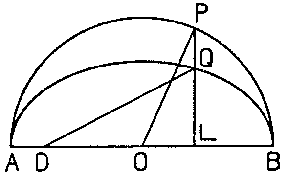# Kepler equation

A transcendental equation of the form

$$y-c\sin y=x.$$

The case $0\leq c<1$ is important for applications; here $y$ is uniquely determined from a given $c$ and $x$. This equation was first considered by J. Kepler (1609) in connection with the problem of planetary motion: Let the ellipse $AQB$ (see Fig.) with focal point $D$ be a planetary orbit, with circumscribed circle $APB$.Figure: k055210a

Then the Kepler equation gives the relation between the eccentric anomaly $y=\angle POA$ and the mean anomaly $x$, $c$ being the eccentricity of the ellipse.

The Kepler equation plays an important role in astronomy in determining the sections of elliptic orbits of planets.